## 主成分分析和聚类¶

• PCA ķÖŹń╗┤
• K-Means
• Ķ┐æķé╗õ╝ĀµÆŁ
• Ķ░▒ĶüÜń▒╗
• ÕćØĶüÜĶüÜń▒╗

### 主成分分析 PCA¶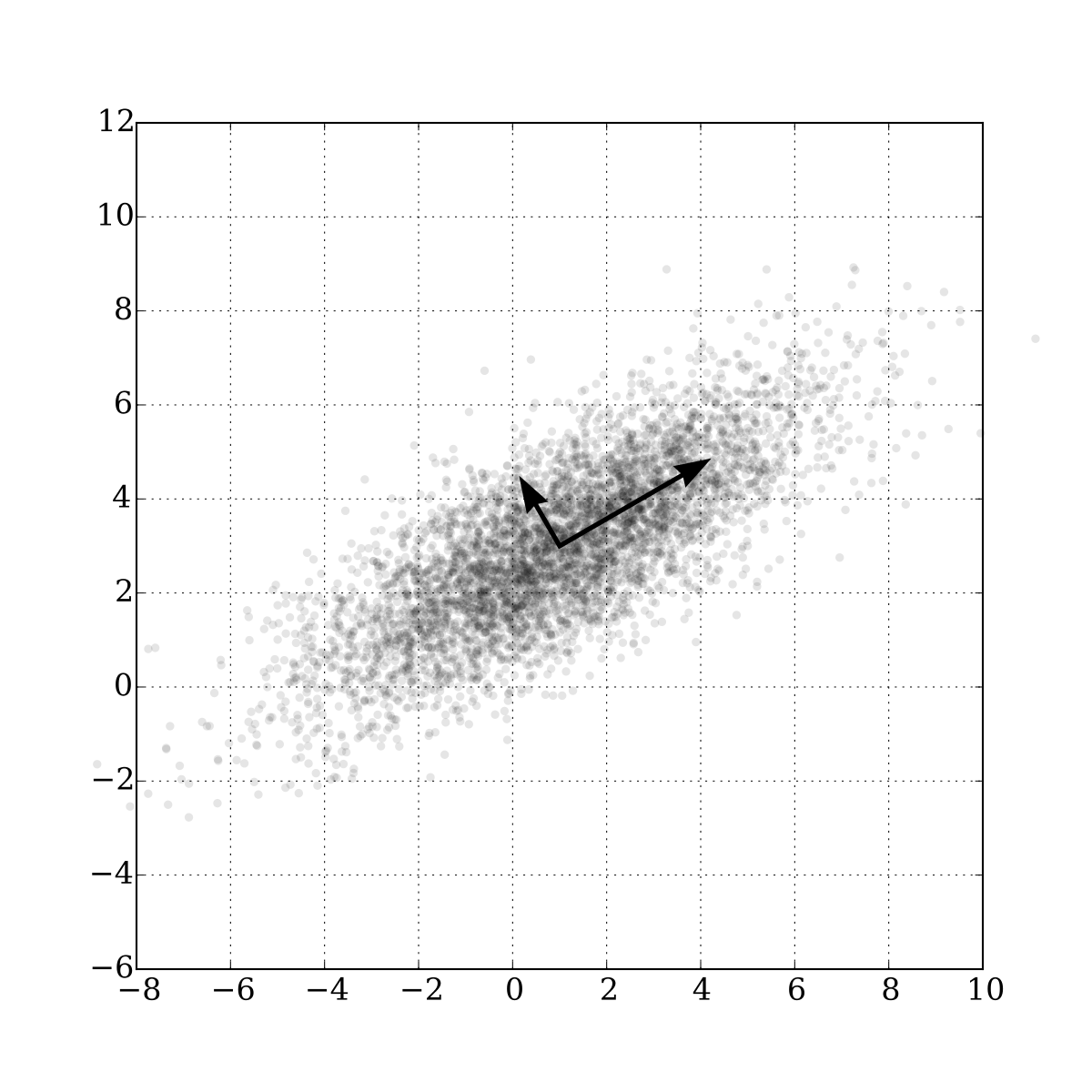õĖ║õ║åµø┤ÕźĮńÜäńÉåĶ¦Ż PCA ńÜäÕĘźõĮ£ÕÄ¤ńÉå’╝īõĖĆĶĄĘµØźń£ŗõĖŖķØóĶ┐ÖÕ╣ģÕøŠŃĆéĶ»Ģµā│õĖĆõĖŗ’╝īÕ”éµ×£Õ░åÕØÉµĀćń│╗õĖŁńÜäµĢ░µŹ«ńé╣µŖĢÕĮ▒Õł░ $x$ ĶĮ┤’╝łµŖĢÕĮ▒Ķ┐ćÕÉÄńÜäµĢ░µŹ«ÕŹ│õĖ║ $x$ ĶĮ┤õĖŖńÜäõĖĆÕĀåµĢ░µŹ«ńé╣’╝ē’╝īµł¢ĶĆģõ╗źÕÉīµĀĘńÜäµ¢╣µ│ĢµŖĢÕĮ▒Õł░ $y$ ĶĮ┤’╝īÕō¬õĖĆõĖ¬µø┤Õ«╣µśōÕī║ÕłåÕæó’╝¤

ÕŠłµśŠńäČµś» $x$ ĶĮ┤ŃĆéÕøĀõĖ║µĢ░µŹ«µŖĢÕĮ▒Õł░ $x$ ĶĮ┤õ╣ŗÕÉÄńøĖµ»öõ║Ä $y$ ĶĮ┤µø┤ÕłåµĢŻ’╝īõ╣¤Õ░▒µś»µĢ░µŹ«µĀĘµ£¼ńÜäµ¢╣ÕĘ«µø┤Õż¦’╝īõ╗ÄĶĆīõ╣¤µø┤Õ«╣µśōÕī║ÕłåŃĆéÕø×Õł░õĖŖÕøŠ’╝īÕ”éµ×£Ķ”üÕ£©ÕØÉµĀćń│╗õĖŁµēŠÕł░õĖĆµĀ╣ĶĮ┤’╝īõĮ┐ÕŠŚµŖĢÕĮ▒Õł░Ķ┐ÖµĀ╣ĶĮ┤ÕÉÄńÜäµĢ░µŹ«ńÜäµ¢╣ÕĘ«µø┤Õż¦’╝īõ╣¤Õ░▒µś»Ķ»┤µø┤ÕłåµĢŻ’╝īÕō¬õĖĆµĀ╣ĶĮ┤µ£ĆÕźĮÕæó’╝¤µśŠńäČ’╝īÕ░▒µś»ÕøŠõĖŁµēĆµĀćńÜäµżŁÕ£åĶĮ┤µ¢╣ÕÉæŃĆé

PCA ńÜäµĆØµā│Õ░▒µś»Ķ┐ÖµĀĘŃĆéÕ”éµ×£Õ░åÕøŠõĖŁńÜäµĢ░µŹ«ķāĮµŖĢÕĮ▒Õł░µżŁÕ£åĶĮ┤õĖŖ’╝īÕłÖµĢ░µŹ«õ╣¤Õ░▒õ╗Äõ║īń╗┤ķÖŹõĖ║õ║åõĖĆń╗┤ŃĆé

õĖ║õ║åÕ░åµĢ░µŹ«ńÜäń╗┤µĢ░õ╗Ä $n$ ń╗┤ķÖŹÕł░ $k$ ń╗┤’╝īµłæõ╗¼µīēńģ¦µĢŻÕ║”’╝łõ╣¤Õ░▒µś»µĢ░µŹ«ńÜäÕłåµĢŻń©ŗÕ║”’╝ēķÖŹõĮÄńÜäķĪ║Õ║ÅÕ»╣ĶĮ┤ÕłŚĶĪ©Ķ┐øĶĪīµÄÆÕ║Å’╝īńäČÕÉÄÕÅ¢Õć║ÕēŹ $k$ ķĪ╣ŃĆé

ńÄ░Õ£©Õ╝ĆÕ¦ŗĶ«Īń«ŚÕÄ¤Õ¦ŗµĢ░µŹ« $n$ ń╗┤ńÜäµĢŻÕ║”ÕĆ╝ÕÆīÕŹÅµ¢╣ÕĘ«ŃĆéµĀ╣µŹ«ÕŹÅµ¢╣ÕĘ«ń¤®ķśĄńÜäÕ«Üõ╣ē’╝īõĖżõĖ¬ńē╣ÕŠüÕłŚńÜäÕŹÅµ¢╣ÕĘ«ń¤®ķśĄĶ«Īń«ŚÕģ¼Õ╝ÅÕ”éõĖŗ’╝Ü

$$cov(X_i, X_j) = E[(X_i - \mu_i) (X_j - \mu_j)] = E[X_i X_j] - \mu_i \mu_j$$

õĖŖÕ╝ÅõĖŁńÜä $\mu_i$ ĶĪ©ńż║ń¼¼ $i$ õĖ¬ńē╣ÕŠüńÜäµ£¤µ£øÕĆ╝ŃĆéĶ┐Öķćīķ£ĆĶ”üµ│©µäÅńÜäµś»’╝īÕŹÅµ¢╣ÕĘ«ķāĮµś»Õ»╣ń¦░ńÜä’╝īĶĆīõĖöÕÉæķćÅõĖÄÕģČĶć¬Ķ║½ńÜäÕŹÅµ¢╣ÕĘ«Õ░▒ńŁēõ║ÄÕģČµĢŻÕ║”ŃĆéÕĮōÕŹÅµ¢╣ÕĘ«õĖ║µŁŻµŚČ’╝īĶ»┤µśÄ $X$ ÕÆī $Y$ µś»µŁŻńøĖÕģ│Õģ│ń│╗’╝øÕŹÅµ¢╣ÕĘ«õĖ║Ķ┤¤µŚČ’╝īĶ»┤µśÄ $X$ ÕÆī $Y$ µś»Ķ┤¤ńøĖÕģ│Õģ│ń│╗’╝øÕŹÅµ¢╣ÕĘ«õĖ║ 0 µŚČ’╝īĶ»┤µśÄ $X$ ÕÆī $Y$ µś»ńøĖõ║Æńŗ¼ń½ŗŃĆé

ÕüćÕ«Ü $\mathbf{X}$ µś»Ķ¦éµĄŗń¤®ķśĄ’╝īÕłÖÕŹÅµ¢╣ÕĘ«ń¤®ķśĄÕ”éõĖŗ’╝Ü

$$\Sigma = E[(\mathbf{X} - E[\mathbf{X}]) (\mathbf{X}-E[\mathbf{X}])^{T}]$$

Õ┐½ķĆ¤Õø×ķĪŠõĖĆõĖŗń¤®ķśĄń¤źĶ»å’╝ÜÕüćÕ«ÜõĖĆõĖ¬ń¤®ķśĄ $M$ ńÜäńē╣ÕŠüÕÉæķćÅõĖ║ $w_i$ õ╗źÕÅŖÕ»╣Õ║öńÜäńē╣ÕŠüÕÉæķćÅõĖ║ $\lambda_i$ ’╝īÕłÖÕ«āõ╗¼õ╝Üµ╗ĪĶČ│õĖŗķØóÕģ¼Õ╝Å’╝Ü

$$M w_i = \lambda_i w_i$$

õĖĆõĖ¬µĀĘµ£¼ $X$ ńÜäÕŹÅµ¢╣ÕĘ«ń¤®ķśĄÕÅ»õ╗źĶĪ©ńż║õĖ║ÕģČĶĮ¼ńĮ«ń¤®ķśĄ $\mathbf{X}^{T}$ õĖÄ $\mathbf{X}$ ńÜäõ╣śń¦»ŃĆéµĀ╣µŹ« ’╝īµĀĘµ£¼ $X$ ńÜäµ£ĆÕż¦µ¢╣ÕĘ«õĮŹõ║ÄÕŹÅµ¢╣ÕĘ«ń¤®ķśĄńÜäµ£ĆÕż¦ńē╣ÕŠüÕĆ╝Õ»╣Õ║öńÜäńē╣ÕŠüÕÉæķćÅõĖŖŃĆéõ╣¤Õ░▒µś»Ķ»┤µā│Ķ”üõ┐ØńĢÖõĖĆõĖ¬ń¤®ķśĄńÜäµ£ĆÕż¦õ┐Īµü»’╝īµłæõ╗¼ÕÅ¬ķ£ĆĶ”üõ┐ØńĢÖĶ»źń¤®ķśĄńÜäµ£ĆÕż¦ńē╣ÕŠüÕĆ╝µēĆÕ»╣Õ║öńÜäńē╣ÕŠüÕÉæķćÅµēĆń╗äµłÉńÜäń¤®ķśĄÕŹ│ÕÅ»’╝īĶ┐ÖõĖ¬Ķ┐ćń©ŗÕ░▒µś»ķÖŹń╗┤õ║åŃĆé

ÕøĀµŁż’╝īõ╗ÄµĢ░µŹ«õĖŁõ┐ØńĢÖńÜäõĖ╗Ķ”üµłÉÕłåÕ░▒µś»õĖÄń¤®ķśĄńÜäķĪČķā© $k$ µ£ĆÕż¦ńē╣ÕŠüÕĆ╝Õ»╣Õ║öńÜäńē╣ÕŠüÕÉæķćÅŃĆé

#### 莺尾花数据集¶

In :
from mpl_toolkits.mplot3d import Axes3D
from sklearn import datasets
from sklearn import decomposition
import numpy as np
import matplotlib.pyplot as plt
import seaborn as sns
import warnings
warnings.filterwarnings('ignore')
sns.set(style='white')
%matplotlib inline
%config InlineBackend.figure_format = 'retina'


ķĆÜĶ┐ć scikit-learn µÅÉõŠøńÜäµĢ░µŹ«ķøåµÄźÕÅŻÕ»╝ÕģźĶÄ║Õ░ŠĶŖ▒µĢ░µŹ«ķøåŃĆé

In :
iris = datasets.load_iris()
X = iris.data
y = iris.target


In :
fig = plt.figure(1, figsize=(6, 5))
plt.clf()
ax = Axes3D(fig, rect=[0, 0, .95, 1], elev=48, azim=134)

plt.cla()

for name, label in [('Setosa', 0), ('Versicolour', 1), ('Virginica', 2)]:
ax.text3D(X[y == label, 0].mean(),
X[y == label, 1].mean() + 1.5,
X[y == label, 2].mean(), name,
horizontalalignment='center',
bbox=dict(alpha=.5, edgecolor='w', facecolor='w'))
# µö╣ÕÅśµĀćńŁŠńÜäķĪ║Õ║Å’╝īĶ«®ÕģČõĖÄµĢ░µŹ«Õī╣ķģŹ
y_clr = np.choose(y, [1, 2, 0]).astype(np.float)
ax.scatter(X[:, 0], X[:, 1], X[:, 2], c=y_clr,
cmap=plt.cm.nipy_spectral)

ax.w_xaxis.set_ticklabels([])
ax.w_yaxis.set_ticklabels([])
ax.w_zaxis.set_ticklabels([])

Out:
[]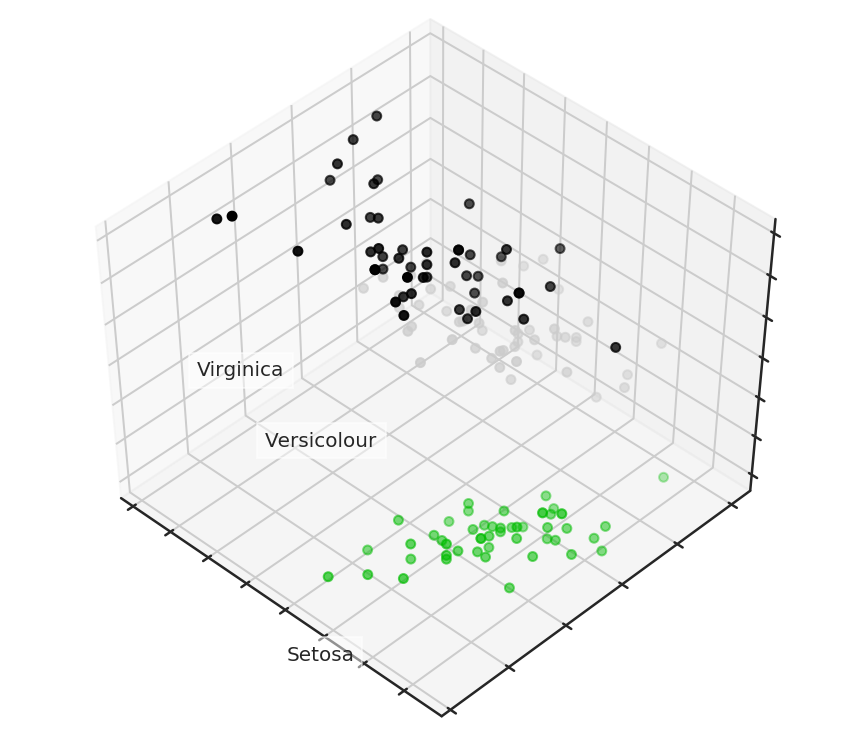In :
from sklearn.tree import DecisionTreeClassifier  # Õ»╝ÕģźÕå│ńŁ¢µĀæµ©ĪÕ×ŗŃĆü
from sklearn.model_selection import train_test_split
from sklearn.metrics import accuracy_score, roc_auc_score  # Ķ»åÕł½ÕćåńĪ«ńÄćĶ«Īń«ŚÕćĮµĢ░


In :
# ÕłÆÕłåĶ«Łń╗āķøåÕÆīµĄŗĶ»Ģķøå
X_train, X_test, y_train, y_test = train_test_split(X, y, test_size=.3,
stratify=y,
random_state=42)

# Õå│ńŁ¢µĀæńÜäµĘ▒Õ║”Ķ«ŠńĮ«õĖ║ 2
clf = DecisionTreeClassifier(max_depth=2, random_state=42)
clf.fit(X_train, y_train)
preds = clf.predict_proba(X_test)
print('Accuracy: {:.5f}'.format(accuracy_score(y_test,
preds.argmax(axis=1))))

Accuracy: 0.88889


In :
# õĮ┐ńö©õ╗Ä sklearn µÅÉõŠøńÜä PCA µÄźÕÅŻ’╝īķÖŹµĢ░µŹ«ķÖŹÕł░ 2 ń╗┤
pca = decomposition.PCA(n_components=2)
X_centered = X - X.mean(axis=0)
pca.fit(X_centered)
X_pca = pca.transform(X_centered)

# ÕÅ»Ķ¦åÕī¢ PCA ķÖŹń╗┤ÕÉÄńÜäń╗ōµ×£
plt.plot(X_pca[y == 0, 0], X_pca[y == 0, 1], 'bo', label='Setosa')
plt.plot(X_pca[y == 1, 0], X_pca[y == 1, 1], 'go', label='Versicolour')
plt.plot(X_pca[y == 2, 0], X_pca[y == 2, 1], 'ro', label='Virginica')
plt.legend(loc=0)

Out:
<matplotlib.legend.Legend at 0x7fdf7a1e0b70>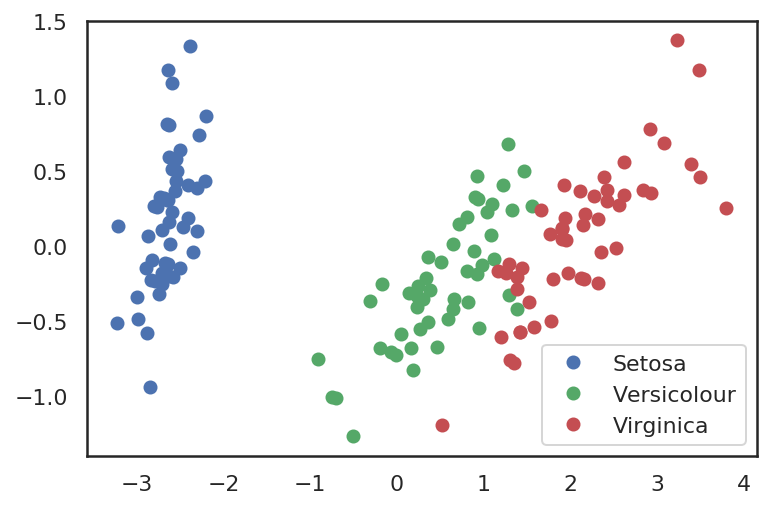In :
# Ķ«Łń╗āķøåÕÉłµĄŗĶ»ĢķøåÕÉīµŚČõĮ┐ńö© PCA Ķ┐øĶĪīķÖŹń╗┤
X_train, X_test, y_train, y_test = train_test_split(X_pca, y, test_size=.3,
stratify=y,
random_state=42)

clf = DecisionTreeClassifier(max_depth=2, random_state=42)
clf.fit(X_train, y_train)
preds = clf.predict_proba(X_test)
print('Accuracy: {:.5f}'.format(accuracy_score(y_test,
preds.argmax(axis=1))))

Accuracy: 0.91111


ķéŻõ╣łķÖŹń╗┤ÕÉÄńÜäµ»ÅõĖ¬õĖ╗µłÉÕłåķāĮµØźĶć¬õ║ÄÕÄ¤Õ¦ŗµĢ░µŹ«ńÜäÕō¬õ║øń╗┤Õ║”Õæó’╝¤Ķ«®µłæõ╗¼ķĆÜĶ┐ćµ¤źń£ŗµ»ÅõĖ¬ń╗┤Õ║”ńÜäµ¢╣ÕĘ«ńÖŠÕłåµ»öµØźĶ¦ŻķćŖĶ┐ÖõĖ¬ķŚ«ķóśŃĆé

In :
for i, component in enumerate(pca.components_):
print("{} component: {}% of initial variance".format(i + 1,
round(100 * pca.explained_variance_ratio_[i], 2)))
print(" + ".join("%.3f x %s" % (value, name)
for value, name in zip(component,
iris.feature_names)))

1 component: 92.46% of initial variance
0.361 x sepal length (cm) + -0.085 x sepal width (cm) + 0.857 x petal length (cm) + 0.358 x petal width (cm)
2 component: 5.31% of initial variance
0.657 x sepal length (cm) + 0.730 x sepal width (cm) + -0.173 x petal length (cm) + -0.075 x petal width (cm)


õ╗ÄõĖŖķØóń╗ōµ×£ÕÅ»ń¤ź’╝īµłæõ╗¼Õ░å 4 ń╗┤ńÜäµĢ░µŹ«ķÖŹõĖ║õ║å 2 ń╗┤µĢ░µŹ«ŃĆéÕ£©ķÖŹń╗┤ÕÉÄńÜäµĢ░µŹ«õĖŁ’╝īń¼¼õĖĆń╗┤’╝łõ╣¤Õ░▒µś»ń¼¼õĖĆõĖ¬µłÉÕłå’╝ēõĖ╗Ķ”üńö▒ÕÄ¤Õ¦ŗµĢ░µŹ«ńÜä $0.361 * \text { sepallength }-0.085 * \text { sepalwidth }+0.857 * \text { petallength }+0.358 * \text { petalwidth }$ ń╗äµłÉŃĆé

#### 手写数字数据集¶

In :
digits = datasets.load_digits()
X = digits.data
y = digits.target


In :
plt.figure(figsize=(14, 5))
for i in range(10):
plt.subplot(2, 5, i + 1)
plt.imshow(X[i, :].reshape([8, 8]), cmap='gray')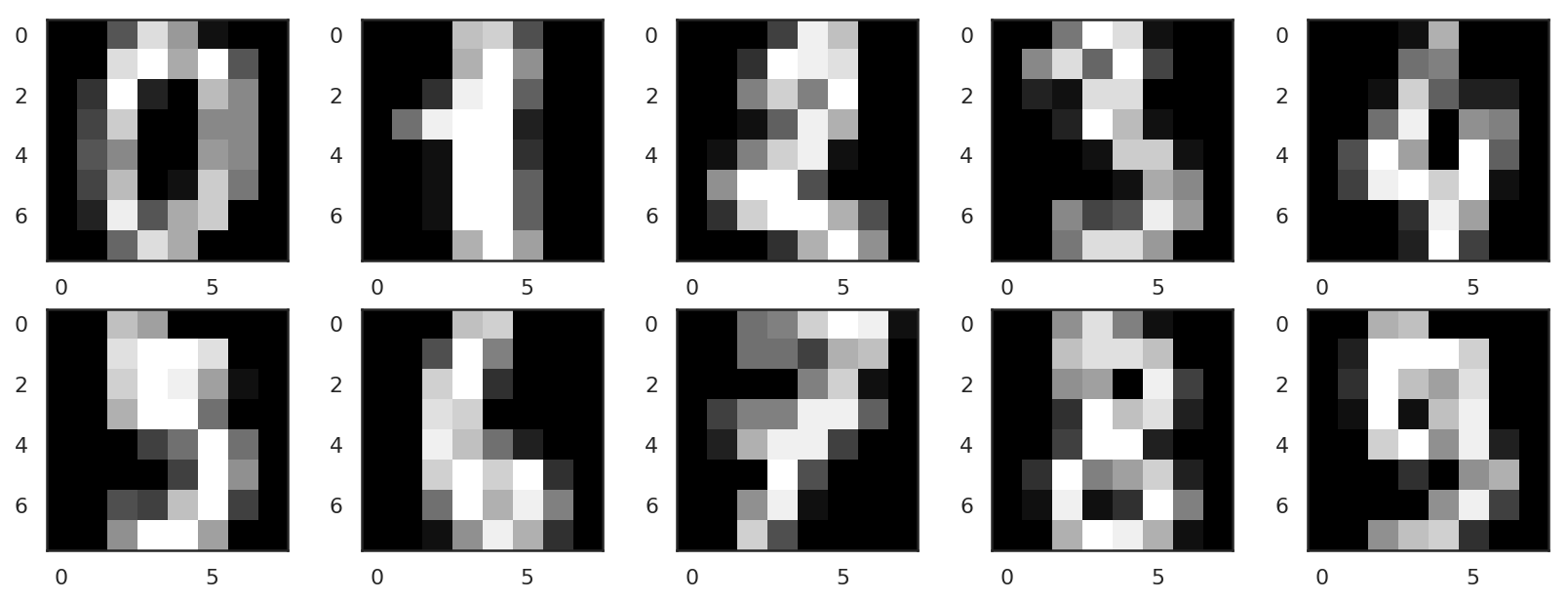µēŗÕåÖµĢ░ÕŁŚõĮōµĢ░µŹ«ķøåńÜäń╗┤Õ║”µś» 8├Ś8 ń╗┤ńÜä’╝īÕŹ│ 64 ń╗┤ŃĆéÕÅ¬µ£ēÕ░åÕģČķÖŹń╗┤ÕćÅÕ░æÕł░ 2 ń╗┤’╝īµēŹĶāĮÕ»╣ÕģČĶ┐øĶĪīÕÅ»Ķ¦åÕī¢’╝īõ╗źõŠ┐µ¤źń£ŗÕģČÕłåÕĖāńŖČµĆüŃĆé

In :
pca = decomposition.PCA(n_components=2)
X_reduced = pca.fit_transform(X)

print('Projecting %d-dimensional data to 2D' % X.shape)

plt.figure(figsize=(6, 5))
plt.scatter(X_reduced[:, 0], X_reduced[:, 1], c=y,
edgecolor='none', alpha=0.7, s=40,
cmap=plt.cm.get_cmap('nipy_spectral', 10))
plt.colorbar()
plt.title('MNIST. PCA projection')

Projecting 64-dimensional data to 2D

Out:
Text(0.5, 1.0, 'MNIST. PCA projection')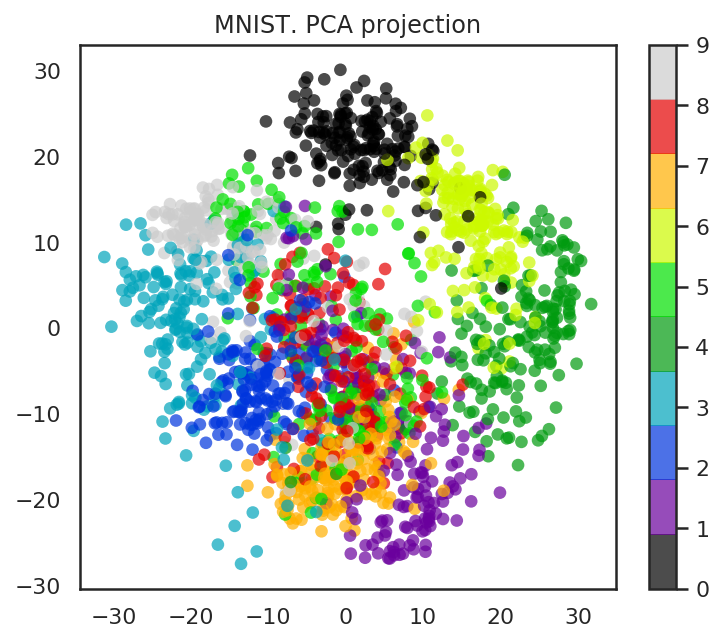In :
from sklearn.manifold import TSNE  # Õ»╝Õģź t-SNE

tsne = TSNE(random_state=17)
X_tsne = tsne.fit_transform(X)

plt.figure(figsize=(6, 5))
plt.scatter(X_tsne[:, 0], X_tsne[:, 1], c=y,
edgecolor='none', alpha=0.7, s=40,
cmap=plt.cm.get_cmap('nipy_spectral', 10))
plt.colorbar()
plt.title('MNIST. t-SNE projection')

Out:
Text(0.5, 1.0, 'MNIST. t-SNE projection')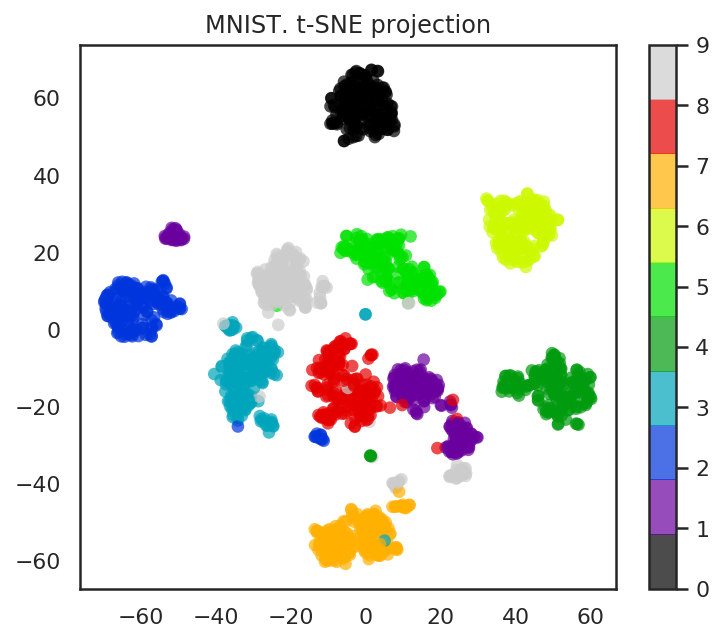In :
pca = decomposition.PCA().fit(X)

plt.figure(figsize=(6, 4))
plt.plot(np.cumsum(pca.explained_variance_ratio_), color='k', lw=2)
plt.xlabel('Number of components')
plt.ylabel('Total explained variance')
plt.xlim(0, 63)
plt.yticks(np.arange(0, 1.1, 0.1))
plt.axvline(21, c='b')
plt.axhline(0.9, c='r')
plt.show()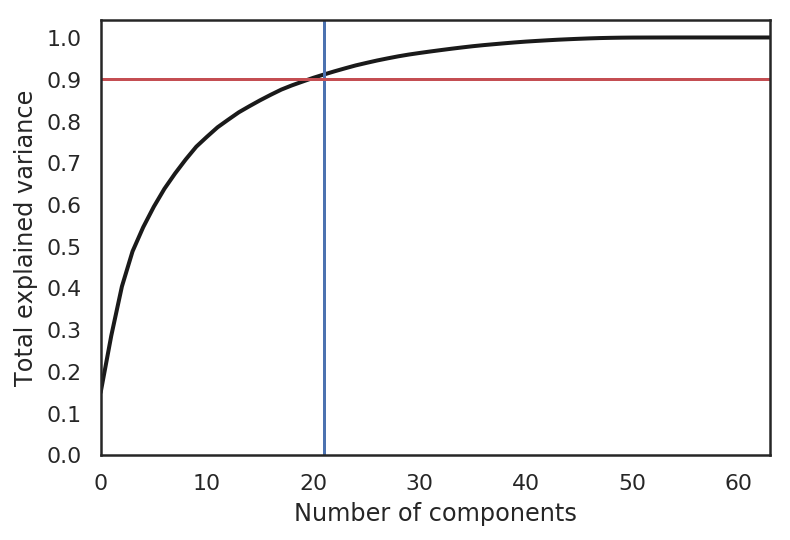õ╗ÄõĖŖÕøŠõĖŁÕÅ»õ╗źń£ŗÕć║’╝īĶ”üµā│õ┐ØńĢÖÕÄ¤Õ¦ŗµĢ░µŹ« 90% ńÜäõ┐Īµü»ŃĆéķ£ĆĶ”üõ┐ØńĢÖ 21 õĖ¬õĖ╗Ķ”üń╗äµłÉµłÉÕłåŃĆéÕøĀµŁż’╝īķ£ĆĶ”üÕ░åń╗┤Õ║”õ╗Ä 64 õĖ¬ńē╣ÕŠüÕćÅÕ░æÕł░ 21 õĖ¬ŃĆé

### 聚类¶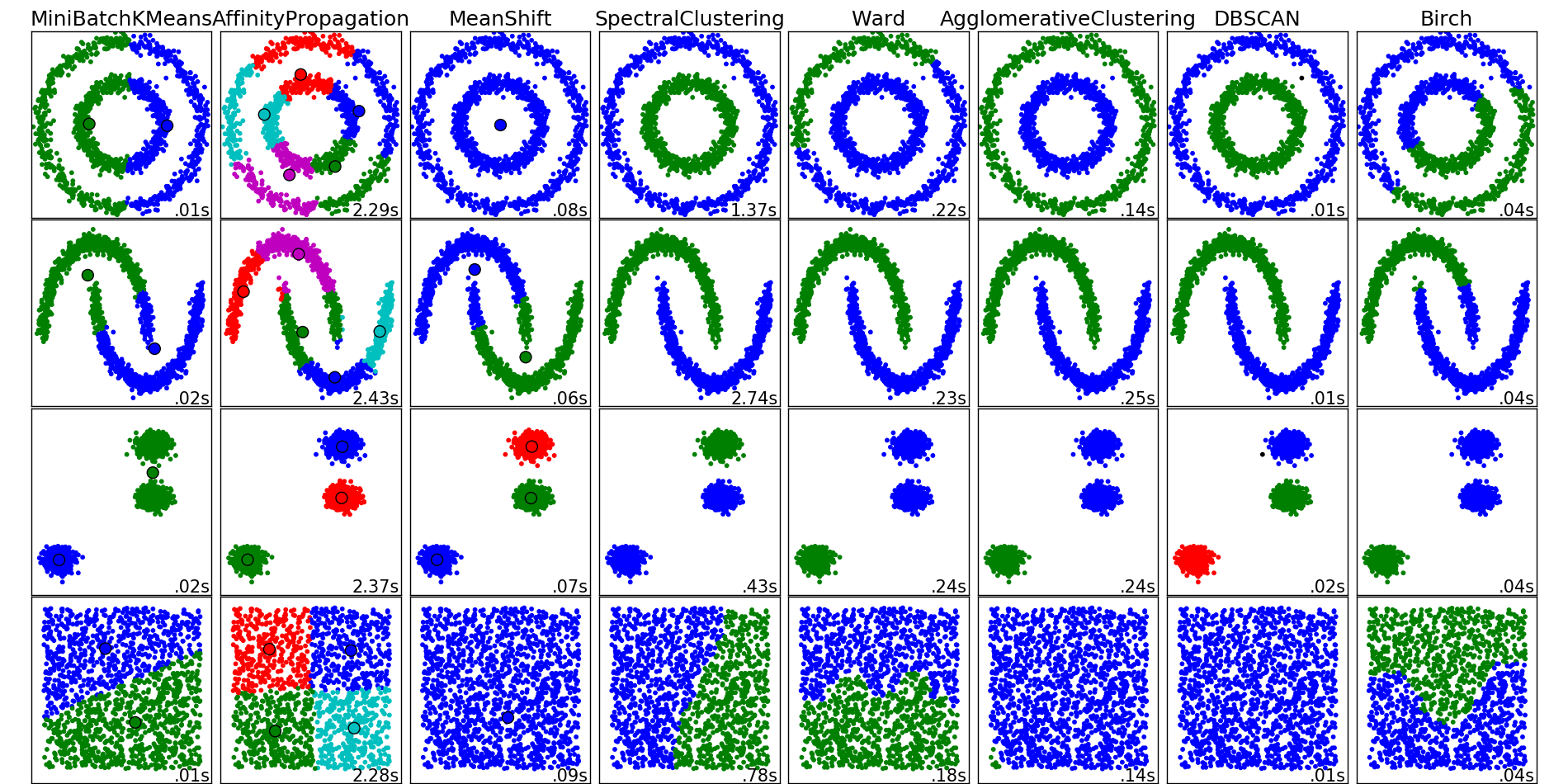õĖŖÕøŠÕ▒Ģńż║ńÜäķāĮµś»µØźĶć¬ scikit-learn ńÜäõĖŹÕÉīń«Śµ│ĢńÜäń╗ōµ×£ńż║õŠŗŃĆé

#### K-Means 聚类¶

K-Means ń«Śµ│Ģµś»µēĆµ£ēĶüÜń▒╗ń«Śµ│ĢõĖŁµ£ĆµĄüĶĪīõĖöµ£Ćń«ĆÕŹĢńÜäń«Śµ│ĢŃĆé õĖŗķØóµś»Õ«āńÜäÕĘźõĮ£ÕÄ¤ńÉå’╝Ü

1. ķĆēµŗ®Ķ«żõĖ║µ£ĆõĮ│ńÜäń▒╗Õł½µĢ░ķćÅ $k$’╝īÕŹ│µĀĘµ£¼Õż¦µ”éÕÅ»õ╗źÕłåõĖ║ÕżÜÕ░æõĖ¬ń░ćŃĆé
2. Õ£©µĢ░µŹ«ń®║ķŚ┤ÕåģķÜÅµ£║ÕłØÕ¦ŗÕī¢ $k$ ńé╣õĖ║ŌĆ£Ķ┤©Õ┐āŌĆØŃĆé

õĖ║õ║åµø┤ÕźĮńÜäńÉåĶ¦Ż K-Means ń«Śµ│ĢńÜäÕÄ¤ńÉå’╝īĶ┐ÖķćīķĆÜĶ┐ćõĖĆõĖ¬õŠŗÕŁÉµØźĶ┐øĶĪīĶ»┤µśÄŃĆéÕģłµ×äÕ╗║Õć║õĖĆõĖ¬µĢ░µŹ«ķøåÕ╣Čńö╗ÕøŠÕ«āńÜäÕłåÕĖāÕøŠ’╝īĶ»źµĢ░µŹ«ķøåÕÉ½µ£ēõĖēõĖ¬ń░ćŃĆé

In :
# µ×äķĆĀÕÅ»ÕłåõĖ║õĖēõĖ¬ń░ćńÜäµĢ░µŹ«
X = np.zeros((150, 2))

np.random.seed(seed=42)
X[:50, 0] = np.random.normal(loc=0.0, scale=.3, size=50)
X[:50, 1] = np.random.normal(loc=0.0, scale=.3, size=50)

X[50:100, 0] = np.random.normal(loc=2.0, scale=.5, size=50)
X[50:100, 1] = np.random.normal(loc=-1.0, scale=.2, size=50)

X[100:150, 0] = np.random.normal(loc=-1.0, scale=.2, size=50)
X[100:150, 1] = np.random.normal(loc=2.0, scale=.5, size=50)

plt.figure(figsize=(5, 5))
plt.plot(X[:, 0], X[:, 1], 'bo')

Out:
[<matplotlib.lines.Line2D at 0x7fdf69c24cc0>]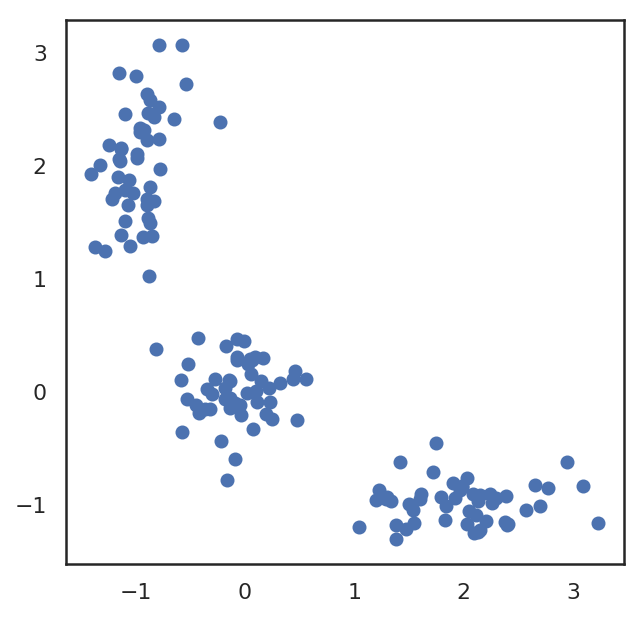In :
# Ķ░āńö© Scipy Õ║ōńÜäĶĘØń”╗Ķ«Īń«ŚÕćĮµĢ░’╝ī
from scipy.spatial.distance import cdist

# ķÜÅµ£║ÕłØÕ¦ŗÕī¢õĖēõĖ¬õĖŁÕ┐āńé╣
np.random.seed(seed=42)
centroids = np.random.normal(loc=0.0, scale=1., size=6)
centroids = centroids.reshape((3, 2))

cent_history = []
cent_history.append(centroids)

for i in range(3):
# Ķ«Īń«Śµ»ÅõĖ¬ńé╣Õł░õĖŁÕ┐āńÜäĶĘØń”╗
distances = cdist(X, centroids)
# ĶÄĘÕÅ¢µĢ░µŹ«Õł½ÕłåÕł░Õō¬õĖ¬ń░ć
labels = distances.argmin(axis=1)
centroids = centroids.copy()
centroids[0, :] = np.mean(X[labels == 0, :], axis=0)
centroids[1, :] = np.mean(X[labels == 1, :], axis=0)
centroids[2, :] = np.mean(X[labels == 2, :], axis=0)

cent_history.append(centroids)


In :
# ÕÅ»Ķ¦åÕī¢ K ÕØćÕĆ╝ĶüÜń▒╗µŁźķ¬ż
plt.figure(figsize=(8, 8))
for i in range(4):
distances = cdist(X, cent_history[i])
labels = distances.argmin(axis=1)

plt.subplot(2, 2, i + 1)
plt.plot(X[labels == 0, 0], X[labels == 0, 1], 'bo', label='cluster #1')
plt.plot(X[labels == 1, 0], X[labels == 1, 1], 'co', label='cluster #2')
plt.plot(X[labels == 2, 0], X[labels == 2, 1], 'mo', label='cluster #3')
plt.plot(cent_history[i][:, 0], cent_history[i][:, 1], 'rX')
plt.legend(loc=0)
plt.title('Step {:}'.format(i + 1))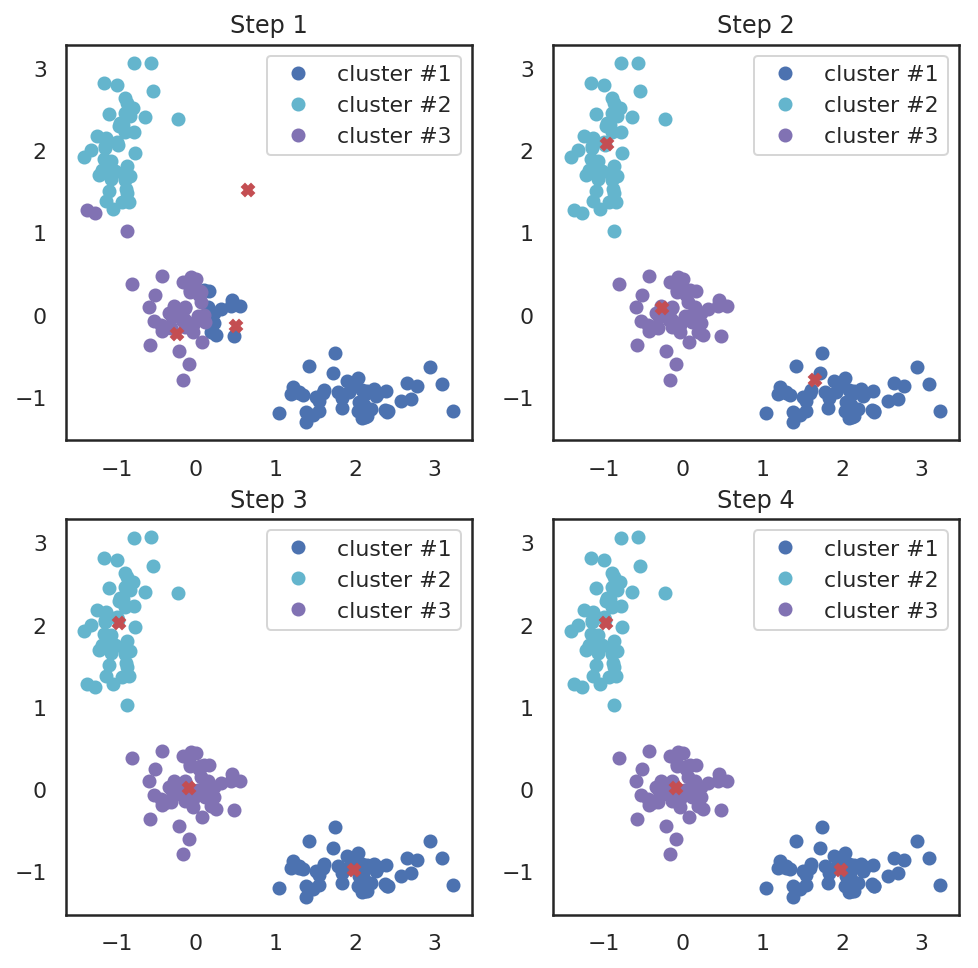#### K-均值算法中 K 值的选择¶

$$J(C) = \sum_{k=1}^K\sum_{i~\in~C_k} ||x_i - \mu_k|| \rightarrow \min\limits_C,$$

Ķ┐ÖķćīńÜä $C_k$ µś»ń¼¼ $k$ õĖ¬ń░ćµēĆµ£ēµĀĘµ£¼ńÜäķøåÕÉł’╝ī$\mu_k$ µś» $C_k$ ńÜäõĖŁÕ┐āŃĆé

õĖŖķØóńÜäÕ«Üõ╣ēń£ŗõ╝╝ÕÉłńÉå’╝īõ╣¤Õ░▒µś»ÕĖīµ£øµĢ░µŹ«ńé╣Õ░ĮÕÅ»ĶāĮµÄźĶ┐æÕ«āõ╗¼µēĆÕ▒×ń░ćńÜäĶ┤©Õ┐āŃĆéõĮåõ╣¤ÕŁśÕ£©õĖĆõĖ¬ķŚ«ķóś’╝īÕĮōĶ┤©Õ┐āµĢ░’╝łõ╣¤Õ░▒µś» K ńÜäÕĆ╝’╝ēńŁēõ║ÄµĀĘµ£¼µĢ░µŚČ’╝īÕģ¼Õ╝ÅÕŠŚÕł░µ£Ćõ╝śĶ¦Ż’╝īÕŹ│ $J(C)$ ĶŠŠÕł░µ£ĆÕ░ÅŃĆéµŁżµŚČńÜäµ»ÅõĖ¬µĀĘµ£¼ķāĮõ╝ÜÕŹĢńŗ¼µŖŖĶć¬ÕĘ▒õĮ£õĖ║õĖĆń▒╗ŃĆéĶ┐ÖµśŠńäČµś»µ▓Īµ£ēµäÅõ╣ēńÜäŃĆéÕøĀõĖ║Ķ┐Öń╗ōµ×£ńøĖÕĮōõ║Äµ▓Īµ£ēĶ┐øĶĪīĶüÜń▒╗ŃĆé

õĖ║õ║åķü┐ÕģŹĶ┐Öń¦ŹµāģÕåĄ’╝īµłæõ╗¼Õ║öĶ»źÕ«Üõ╣ēõĖĆõĖ¬ÕćĮµĢ░’╝īõĮ┐ÕŠŚ $J(C)$ õĖŗķÖŹÕŠŚõĖŹķéŻõ╣łÕ┐½ŃĆéõĮ┐ńö©Õģ¼Õ╝ÅµÅÅĶ┐░Õ”éõĖŗ’╝Ü

$$D(k) = \frac{|J(C_k) - J(C_{k+1})|}{|J(C_{k-1}) - J(C_k)|} \rightarrow \min\limits_k$$

In :
from sklearn.cluster import KMeans  # Õ»╝Õģź K-ÕØćÕĆ╝ĶüÜń▒╗µ©ĪÕ×ŗ


µ▒éÕć║ K ÕĆ╝ÕŠŚķĆēµŗ®õĖÄ $J(C_k)$ ńÜäÕģ│ń│╗’╝īÕ╣Čńö╗Õć║Õ«āõ╗¼ńÜäÕģ│ń│╗ÕøŠŃĆé

In :
inertia = []
for k in range(1, 8):
kmeans = KMeans(n_clusters=k, random_state=1).fit(X)
inertia.append(np.sqrt(kmeans.inertia_))
plt.plot(range(1, 8), inertia, marker='s')
plt.xlabel('$k$')
plt.ylabel('$J(C_k)$')

Out:
Text(0, 0.5, '$J(C_k)$')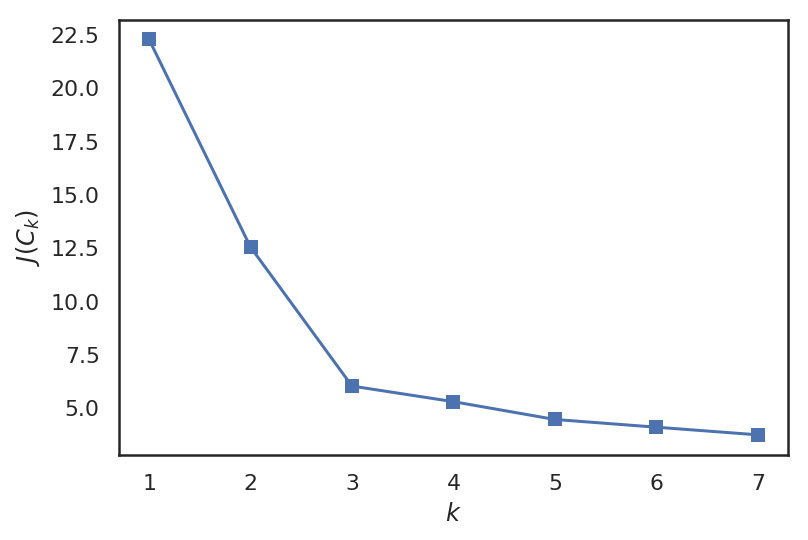õ╗ÄõĖŖÕøŠõĖŁ’╝īÕÅ»õ╗źń£ŗÕł░ÕĮō $k$ Õ░Åõ║Ä 3 µŚČ’╝ī $J(C_k)$ õĖŗķÖŹÕŠŚķØ×ÕĖĖÕ┐½ŃĆéõ╣ŗÕÉÄńøĖÕ»╣Õ╣│ń©│ŃĆéĶ┐ÖµäÅÕæ│ńØĆķĆēµŗ® $k$ ńŁēõ║Ä 3 µ£ĆõĖ║ÕÉłķĆéŃĆé

#### K-均值存在的问题¶

Õ«×ķÖģõĖŖ’╝īK-Means ń«Śµ│Ģµś»õĖĆõĖ¬ $\text{NPhard}$ ķŚ«ķóśŃĆéÕ»╣õ║Ä $n$ õĖ¬ $d$ ń╗┤µĢ░µŹ«’╝īÕĮōµłæõ╗¼µā│Ķ”üÕ░åÕģČĶüÜõĖ║ $k$ õĖ¬ń░ćµŚČ’╝īK-Means ń«Śµ│ĢńÜäÕżŹµØéÕ║”õĖ║ $O(n^{d k+1})$ ’╝īĶ┐ÖµäÅÕæ│ńØĆµ©ĪÕ×ŗńÜäĶ«Łń╗āķ£ĆĶ”üÕż¦ķćÅńÜäµŚČķŚ┤ŃĆéõĖŹĶ┐ć’╝īĶ┐Öķćīµ£ēõĖĆõ║øÕÉ»ÕÅæÕ╝Åµ¢╣µ│ĢÕÅ»õ╗źń╝ōĶ¦ŻĶ┐ÖõĖ¬ķŚ«ķóś’╝īõŠŗÕ”éÕāÅ MiniBatch K-Means ń«Śµ│ĢŃĆé Õ«āµ»Åµ¼Īõ╗ģķććńö©ķā©ÕłåµĢ░µŹ«ĶĆīõĖŹµś»õĖĆµ¼ĪõĮ┐ńö©µĢ┤õĖ¬µĢ░µŹ«ķøå’╝īńäČÕÉÄķĆÜĶ┐ćÕēŹķØóµēĆĶ┐░ńÜäµ¢╣µ│Ģ’╝īķĆÜĶ┐ćõĖĆõĖ¬ń░ćõĖŁµēĆµ£ēĶ¦éµĄŗńé╣ńÜäÕ╣│ÕØćÕĆ╝µØźń¦╗ÕŖ©Ķ┤©Õ┐āŃĆé

Õģ│õ║Ä K-Means ÕÆī MiniBatch K-Means ńÜäÕī║Õł½µł¢ÕĘ«Õ╝éÕÅ»õ╗źµ¤źķśģĶ┐Öõ╗Į ŃĆé

#### 近邻传播算法¶

Ķ┐æķé╗õ╝ĀµÆŁõ╣¤Õ▒×õ║ÄĶüÜń▒╗ń«Śµ│ĢõĖŁńÜäõĖĆń¦ŹŃĆé õĖÄ K-Means õĖŹÕÉīńÜäµś»’╝īĶ┐Öń¦Źµ¢╣µ│ĢõĖŹķ£ĆĶ”üõ║ŗÕģłĶ«ŠńĮ«ń░ćńÜäµĢ░ķćÅ’╝īõ╣¤Õ░▒µś» K ńÜäÕĆ╝ŃĆé Ķ┐æķé╗õ╝ĀµÆŁń«Śµ│ĢńÜäõĖ╗Ķ”üµĆØµā│µś»µĀ╣µŹ«Ķ¦éµĄŗńé╣õ╣ŗķŚ┤ńÜäńøĖõ╝╝µĆ¦µØźÕ»╣µĢ░µŹ«Ķ┐øĶĪīĶüÜń▒╗ŃĆé

Õ”éµ×£Ķ¦éÕ»¤ $x_i$ õĖÄĶ¦éÕ»¤ńé╣ $x_y$ ńøĖõ╝╝’╝īõĮåõĖÄĶ¦éÕ»¤ńé╣ $x_k$ õĖŹńøĖõ╝╝’╝īķéŻõ╣łÕÅ»õ╗źÕ«Üõ╣ēõĖĆõĖ¬ńøĖõ╝╝Õ║”ÕćĮµĢ░ $s$’╝īõĮ┐ÕŠŚ $s(x_i, x_j) > s(x_i, x_k)$ ŃĆéķĆÜÕĖĖķććńö©ĶĘØń”╗ńÜäĶ┤¤Õ╣│µ¢╣ $s(x_i, x_j) = - ||x_i - x_j||^{2}$ µØźÕ║”ķćÅõĖżõĖ¬Ķ¦éµĄŗńé╣ńÜäńøĖõ╝╝µĆ¦ŃĆé

õĖ║õ║åõŠ┐õ║ÄĶ«Īń«Ś’╝īķĆÜÕĖĖķĆÜĶ┐ćÕ«Üõ╣ēõĖżõĖ¬ń¤®ķśĄµØźµÅÅĶ┐░Ķ┐Öń¦ŹŌĆ£ńøĖõ╝╝µĆ¦ŌĆØ’╝īÕłåÕł½µś» $r_{i,k}$ ÕÆī $a_{i,k}$ŃĆé $r_{i,k}$ ĶĪ©ńż║ÕÉĖÕ╝ĢÕ║”’╝īÕŹ│µĀĘµ£¼ $x_k$ ķĆéÕÉłõĮ£õĖ║ $x_i$ ńÜäĶüÜń▒╗õĖŁńÜäńÜäń©ŗÕ║”ŃĆé $a_{i,k}$ ĶĪ©ńż║ÕĮÆÕ▒×Õ║”’╝īõ╣¤Õ░▒µś»µĀĘµ£¼ $x_i$ ķĆēµŗ® $x_k$ õĮ£õĖ║ĶüÜń▒╗õĖŁÕ┐āńÜäÕÉłķĆéń©ŗÕ║”ŃĆéÕ«āõ╗¼ńÜäÕģ¼Õ╝ÅĶĪ©ĶŠŠÕ”éõĖŗ’╝Ü

$$r_{i,k} \leftarrow s_(x_i, x_k) - \max_{k' \neq k} \left\{ a_{i,k'} + s(x_i, x_k') \right\}$$
$$a_{i,k} \leftarrow \min \left( 0, r_{k,k} + \sum_{i' \not\in \{i,k\}} \max(0, r_{i',k}) \right), \ \ \ i \neq k$$
$$a_{k,k} \leftarrow \sum_{i' \neq k} \max(0, r_{i',k})$$

#### 谱聚类¶

$$A_{i, j} = - ||x_i - x_j||^{2}$$

#### 凝聚聚类¶

1. ķ”¢ÕģłÕ░åµ»ÅõĖ¬Ķ¦éµĄŗńé╣ķāĮõĮ£õĖ║õĖĆõĖ¬ń░ć
2. ńäČÕÉÄµīēķÖŹÕ║ÅÕ»╣µ»ÅõĖżõĖ¬ń░ćõĖŁÕ┐āõ╣ŗķŚ┤ĶĘØń”╗Ķ┐øĶĪīµÄÆÕ║Å
3. ÕÅ¢µ£ĆĶ┐æńÜäõĖżõĖ¬ńøĖķé╗ńÜäń░ćÕ╣ČÕ░åÕ«āõ╗¼ÕÉłÕ╣ČõĖ║õĖĆõĖ¬ń░ć’╝īńäČÕÉÄķćŹµ¢░Ķ«Īń«Śń░ćõĖŁÕ┐ā
4. ķćŹÕżŹµŁźķ¬ż 2 ÕÆī 3 ’╝īńø┤Õł░µēĆµ£ēĶ¦éµĄŗńé╣ķāĮÕÉłÕ╣ČÕł░õĖĆõĖ¬ń░ćõĖŁ

1. ÕŹĢķōŠµÄź $$d(C_i, C_j) = min_{x_i \in C_i, x_j \in C_j} ||x_i - x_j||$$
2. Õģ©Ķ┐×µÄź $$d(C_i, C_j) = max_{x_i \in C_i, x_j \in C_j} ||x_i - x_j||$$
3. Õ╣│ÕØćĶ┐×µÄź $$d(C_i, C_j) = \frac{1}{n_i n_j} \sum_{x_i \in C_i} \sum_{x_j \in C_j} ||x_i - x_j||$$
4. Ķ┤©Õ┐āĶ┐×µÄź $$d(C_i, C_j) = ||\mu_i - \mu_j||$$

In :
from scipy.cluster import hierarchy
from scipy.spatial.distance import pdist

X = np.zeros((150, 2))
# µ×äÕ╗║µĢ░µŹ«ķøå
np.random.seed(seed=42)
X[:50, 0] = np.random.normal(loc=0.0, scale=.3, size=50)
X[:50, 1] = np.random.normal(loc=0.0, scale=.3, size=50)

X[50:100, 0] = np.random.normal(loc=2.0, scale=.5, size=50)
X[50:100, 1] = np.random.normal(loc=-1.0, scale=.2, size=50)

X[100:150, 0] = np.random.normal(loc=-1.0, scale=.2, size=50)
X[100:150, 1] = np.random.normal(loc=2.0, scale=.5, size=50)

# pdistÕ░åĶ«Īń«ŚµłÉÕ»╣ĶĘØń”╗ń¤®ķśĄńÜäõĖŖõĖēĶ¦ÆÕĮó
distance_mat = pdist(X)
# Ķ┐×µÄź - µś»õĖĆń¦ŹÕćØĶüÜń«Śµ│ĢńÜäÕ«×ńÄ░
plt.figure(figsize=(10, 5))
dn = hierarchy.dendrogram(Z, color_threshold=0.5)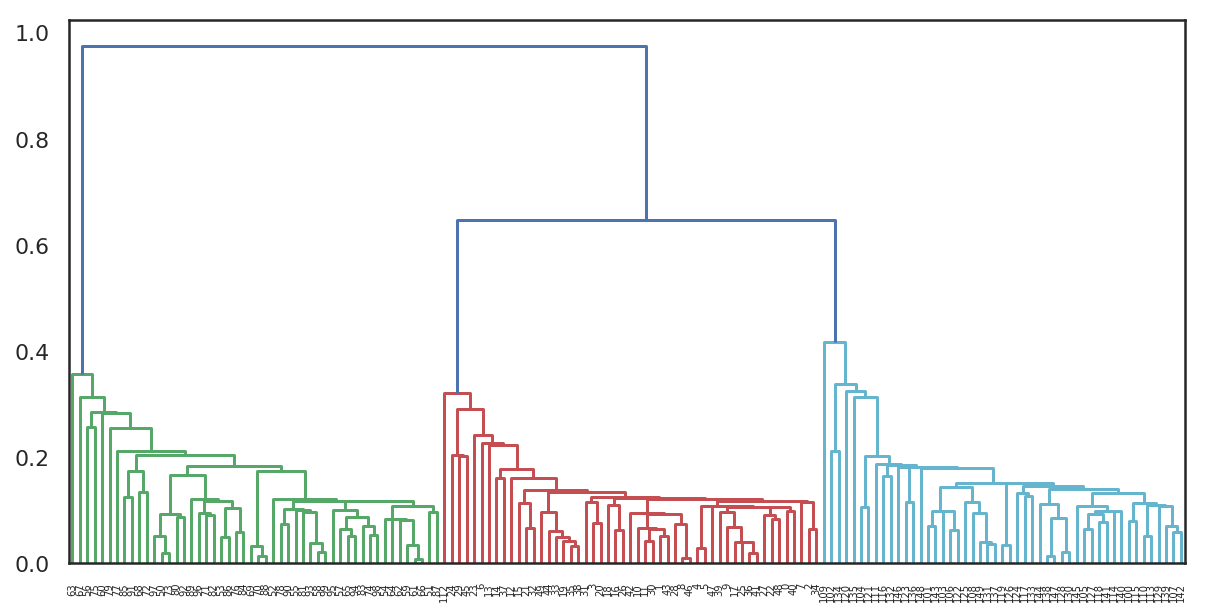### 聚类模型评价¶

#### 调整兰德指数¶

Õģ░ÕŠĘµīćµĢ░µś»õĖĆń¦ŹÕĖĖńö©ńÜäĶüÜń▒╗Ķ»äõ╗Ęµ¢╣µ│ĢŃĆéÕ£©Ķ»źĶ»äõ╗Ęµ¢╣µ│ĢõĖŁ’╝īķ£ĆĶ”üń¤źķüōµĢ░µŹ«ńÜäń£¤Õ«×µĀćńŁŠ’╝īõ╣¤ķ£ĆĶ”üµĢ░µŹ«ķøåńÜäĶüÜń▒╗ń╗ōµ×£ŃĆé Ķ«Š $n$ µś»µĀĘµ£¼õĖŁµĢ░µŹ«ńé╣Õ»╣µĢ░ńÜäµĢ░ķćÅŃĆé Ķ«Š $a$ ĶĪ©ńż║Õ£©ń£¤Õ«×µĀćńŁŠõĖÄĶüÜń▒╗ń╗ōµ×£õĖŁķāĮµś»ÕÉīń▒╗Õł½ńÜäĶ¦éµĄŗńé╣Õ»╣µĢ░ŃĆé $b$ ĶĪ©ńż║Õ£©ń£¤Õ«×µĀćńŁŠõĖÄĶüÜń▒╗ń╗ōµ×£õĖŁķāĮµś»õĖŹÕÉīń▒╗Õł½ńÜäĶ¦éµĄŗńé╣Õ»╣µĢ░ŃĆéÕģ░ÕŠĘµīćµĢ░ÕÅ»õ╗źĶĪ©ńż║õĖ║’╝Ü

$$\text{RI} = \frac{2(a + b)}{n(n-1)}.$$

µŹóÕÅźĶ»ØĶ»┤’╝īÕģ░ÕŠĘµīćµĢ░Ķ»äõ╝░ÕłåÕē▓ÕÉÄńÜäĶüÜń▒╗ń╗ōµ×£ÕÆīÕłØÕ¦ŗµĀćńŁŠõĖĆĶć┤ńÜäµ»öõŠŗŃĆéÕ»╣õ║ÄķÜÅµ£║ń╗ōµ×£’╝ī$\text{RI}$ Õ╣ČõĖŹĶāĮõ┐ØĶ»üÕłåµĢ░µÄźĶ┐æ 0 ŃĆéõĖ║õ║åÕ«×ńÄ░Õ£©ĶüÜń▒╗ń╗ōµ×£µś»ķÜÅµ£║õ║¦ńö¤ńÜäµāģÕåĄõĖŗ’╝īÕģ░ÕŠĘµīćµĢ░Õ║öĶ»źµÄźĶ┐æķøČ’╝īµēĆõ╗źµ£ēÕ┐ģĶ”üń╝®µöŠÕģČÕż¦Õ░Å’╝īńö▒µŁżÕŠŚÕł░õ║åĶ░āµĢ┤Õģ░ÕŠĘµīćµĢ░’╝Ü

$$\text{ARI} = \frac{\text{RI} - E[\text{RI}]}{\max(\text{RI}) - E[\text{RI}]}.$$

Ķ»źĶ»äõ╗Ęµ¢╣µ│Ģµś»Õ»╣ń¦░ńÜä’╝īõĖŹõŠØĶĄ¢õ║ÄµĀćńŁŠµÄÆÕłŚķĪ║Õ║ÅŃĆé ÕøĀµŁż’╝īĶ»źµīćµĢ░µś»µĀĘµ£¼õĖŹÕÉīÕłÆÕłåõ╣ŗķŚ┤ĶĘØń”╗ńÜäÕ║”ķćÅŃĆé $\text{ARI}$ ÕÅ»ÕÅ¢ $[-1,1]$ ĶīāÕø┤ÕåģńÜäÕĆ╝ŃĆé ÕģČÕĆ╝ĶČŖÕż¦ĶĪ©ńż║ĶüÜń▒╗ń╗ōµ×£ĶČŖÕźĮŃĆé

#### 调整互信息¶

Ķ»źĶ»äõ╗ĘµīćµĀćõĖÄ $\text{ARI}$ ń▒╗õ╝╝ŃĆé Õ«āõ╣¤µś»Õ»╣ń¦░ńÜä’╝īõĖŹõŠØĶĄ¢õ║ÄµĀćńŁŠńÜäÕģĘõĮōÕĆ╝ÕÆīµÄÆÕłŚķĪ║Õ║ÅŃĆé õ║Æõ┐Īµü» $\text{MI}$ ńö▒ ÕćĮµĢ░Õ«Üõ╣ē’╝īńö©µØźĶĪĪķćÅń£¤Õ«×µĢ░µŹ«ÕłåÕĖāõĖÄĶüÜń▒╗ń╗ōµ×£ÕłåÕĖāńÜäÕÉ╗ÕÉłń©ŗÕ║”ŃĆé

ÕÉī $\text{ARI}$ õĖĆµĀĘ’╝īĶ┐Öķćīõ╣¤Õ«Üõ╣ēõ║åĶ░āµĢ┤õ║Æõ┐Īµü» $\text{AMI}$ ŃĆé Ķ┐ÖÕÅ»õ╗źķü┐ÕģŹķÜÅńØĆĶüÜń▒╗µĢ░ķćÅńÜäÕó×ÕŖĀĶĆīÕ»╝Ķć┤ $\text{MI}$ µīćµĢ░Õó×ķĢ┐ŃĆé$\text{AMI}$ õĮŹõ║Ä $[0,1]$ ĶīāÕø┤ÕåģŃĆé ÕģČÕĆ╝ĶČŖÕż¦µäÅÕæ│ńØĆĶüÜń▒╗ń╗ōµ×£ĶČŖÕźĮŃĆé

#### 同质性、完整性、V-mearure¶

õ╗ÄÕĮóÕ╝ÅõĖŖµØźń£ŗ’╝īĶ┐Öõ║øµīćµĀćõ╣¤µś»Õ¤║õ║ÄńåĄÕćĮµĢ░ÕÆīµØĪõ╗ČńåĄÕćĮµĢ░µØźÕ«Üõ╣ēńÜä’╝īÕ░åĶüÜń▒╗ń╗ōµ×£ń£ŗõĮ£µś»ń”╗µĢŻÕłåÕĖā’╝Ü

$$h = 1 - \frac{H(C\mid K)}{H(C)}, c = 1 - \frac{H(K\mid C)}{H(K)}$$

ÕģČõĖŁ $K$ µś»ĶüÜń▒╗ń╗ōµ×£’╝ī$C$ µś»ÕÄ¤Õ¦ŗµĢ░µŹ«ŃĆé ÕøĀµŁż’╝ī$h$ Ķ»äõ╝░ńÜäµś»µ»ÅõĖ¬ń░ćµś»ÕÉ”ńö▒ńøĖÕÉīń▒╗Õł½ńÜäµĢ░µŹ«ńé╣ń╗äµłÉ’╝ī$c$ Ķ»äõ╝░ńÜäµś»ńøĖÕÉīń▒╗Õł½ńÜäµĢ░µŹ«ńé╣õĖÄµēĆÕ▒×ń░ćńÜäÕī╣ķģŹń©ŗÕ║”ŃĆé Ķ┐Öõ║øµīćµĀćõĖŹµś»Õ»╣ń¦░ńÜäŃĆéõĖżĶĆģķāĮõĮŹõ║Ä $[0,1]$ ĶīāÕø┤Õåģ’╝īĶĆīµÄźĶ┐æ $1$ ńÜäÕĆ╝ĶĪ©ńż║µø┤ÕćåńĪ«ńÜäĶüÜń▒╗ń╗ōµ×£ŃĆé Ķ┐Öõ║øµīćµĀćńÜäÕĆ╝õĖŹÕāÅ $\text{ARI}$ µł¢ $\text{AMI}$ µīćµĀćĶ┐øĶĪīń╝®µöŠĶ┐ć’╝īÕøĀµŁżÕÅ¢Õå│õ║Äń░ćńÜäµĢ░ķćÅŃĆé

ÕĮōń░ćńÜäµĢ░ķćÅÕŠłÕż¦õĖöĶ¦éµĄŗńé╣ńÜäµĢ░ķćÅÕŠłÕ░ÅµŚČ’╝īĶ»źĶ»äõ╗Ęµ¢╣µ│Ģõ╣¤õĖŹõ╝ÜõĮ┐Ķ»äõ╝░ÕĆ╝µÄźĶ┐æķøČŃĆé Õ£©Ķ┐Öń¦ŹµāģÕåĄõĖŗ’╝īõĮ┐ńö© $\text{ARI}$ ÕÅ»ĶāĮõ╝Üµø┤ÕÉłńÉåŃĆé õĮåµś»’╝īÕ”éµ×£Ķ¦éµĄŗńé╣ĶČģĶ┐ć 100 õĖ¬’╝īõĖöń░ćńÜäµĢ░ķćÅÕ░æõ║Ä 10 õĖ¬’╝īµŁżķŚ«ķóśÕłÖÕÅ»õ╗źÕ┐ĮńĢźŃĆé

$V-mearure$ ń╗ōÕÉłõ║å $h$ ÕÆī $c$’╝īõĖ║ $h$ ÕÆī $c$ ńÜäĶ░āÕÆīÕ╣│ÕØćÕĆ╝ŃĆéńö©µØźĶĪĪķćÅõĖżõĖ¬ÕłÆÕłåń╗ōµ×£ńÜäõĖĆĶć┤µĆ¦ŃĆéÕģČÕģ¼Õ╝ÅÕ”éõĖŗ’╝Ü

$$v = 2\frac{hc}{h+c}$$

#### 轮廓系数¶

õĖÄõĖŖĶ┐░Ķ»äõ╗ĘµīćµĀćńøĖÕÅŹ’╝īĶĮ«Õ╗ōń│╗µĢ░Õ╣ČõĖŹķ£ĆĶ”üÕģ│õ║ÄÕÄ¤Õ¦ŗµĢ░µŹ«ńÜäń£¤Õ«×µĀćńŁŠõ┐Īµü»ŃĆéõ╗ģõĮ┐ńö©ĶüÜń▒╗ń╗ōµ×£µØźõ╝░Ķ«ĪĶüÜń▒╗ńÜäĶ┤©ķćÅŃĆéĶ«Š $a$ µś»µĢ░µŹ«ńé╣õĖÄõĖĆõĖ¬ń░ćÕåģÕģČõ╗¢Ķ¦éµĄŗńé╣õ╣ŗķŚ┤ĶĘØń”╗ńÜäÕ╣│ÕØćÕĆ╝’╝ī$b$ µś»Ķ¦éµĄŗńé╣Õł░µ£ĆĶ┐æń░ćńÜäĶ¦éµĄŗńé╣ńÜäÕ╣│ÕØćĶĘØń”╗ŃĆéÕłÖõĖĆõĖ¬Ķ¦éµĄŗńé╣ńÜäĶĮ«Õ╗ōÕĆ╝õĖ║’╝Ü

$$s = \frac{b - a}{\max(a, b)}$$

µĀĘµ£¼ńÜäĶĮ«Õ╗ōÕĆ╝µś»µēĆµ£ēµĀĘµ£¼ĶĮ«Õ╗ōÕĆ╝ńÜäÕ╣│ÕØćÕĆ╝ŃĆéĶ»źń│╗µĢ░ńÜäÕÅ¢ÕĆ╝ĶīāÕø┤õĖ║ $[- 1,1]$ŃĆéĶĮ«Õ╗ōń│╗µĢ░ÕĆ╝ĶČŖķ½ś’╝īĶĪ©ńż║ĶüÜń▒╗ńÜäń╗ōµ×£ĶČŖÕźĮŃĆé

ÕøĀµŁż’╝īķĆÜÕĖĖÕ”éµ×£õĖŹń¤źķüōµĢ░µŹ«ÕÅ»õ╗źÕłåõĖ║ÕćĀń▒╗’╝īÕłÖÕÅ»õ╗źķĆÜĶ┐ćĶÄĘÕÅ¢µ£ĆÕż¦Õī¢ĶĮ«Õ╗ōń│╗µĢ░µØźńĪ«Õ«Üµ£ĆõĮ│ĶüÜń▒╗µĢ░ $k$ŃĆé

õĖĆĶł¼µāģÕåĄõĖŗ’╝īķāĮõĖŹķ£ĆĶ”üµłæõ╗¼ÕÄ╗Õ«×ńÄ░õĖŖĶ┐░ńÜäĶ»äõ╗Ęµ¢╣µ│Ģ’╝īÕøĀõĖ║Õ£© sklearn.metrics µÄźÕÅŻõĖŁÕĘ▓ń╗ÅµÅÉõŠø’╝īÕÅ¬ķ£ĆÕ»╝ÕģźÕŹ│ÕÅ»ŃĆé

µ£ĆÕÉÄ’╝īķĆÜĶ┐ćÕ«×ķ¬īµØźń£ŗń£ŗĶ┐Öõ║øµīćµĀćµś»Õ”éõĮĢĶ»äõ╗Ę MNIST µēŗÕåÖµĢ░ÕŁŚµĢ░µŹ«ķøåńÜä’╝īÕģ